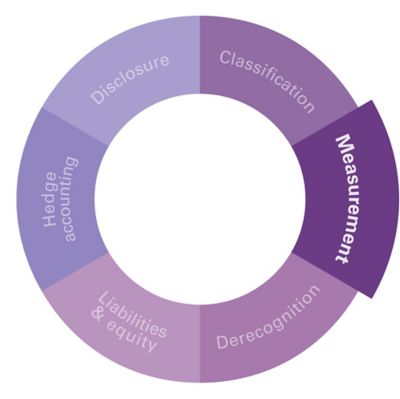AASB 9 FINANCIAL INSTRUMENTS Q&A SERIES

The accounting for unamortised transaction costs differs depending on whether a loan modification is substantial or not. In this article, KPMG explains how to deal with unamortised transaction costs for different types of modifications.## Scenario

Company P has a \$10 million bank loan with the following key terms at inception:

• 6 year maturity
• 7% p.a. fixed interest, and
• Company P can prepay the loan at any time without significant penalty.

After 1 year, Company P successfully renegotiates the terms of its loan to reduce the contractual interest rate from 7% to 5% p.a. to reflect current market interest rates.

When Company P obtained the initial loan, it incurred the following costs:

Type of costs incurred Total
amount
Remaining unamortised
portion after 1 year
Legal costs \$13,000 \$11,196
Total \$24,000 \$20,669

Inclusive of the above transaction costs, the effective interest rate determined on initial recognition was 7.05% (comprising the contractual rate of 7% and an adjustment for transaction costs of 0.05%)

The carrying amount of the loan, based on the present value of the remaining cash flows of the original loan, at date of modification is \$9,979,331.

QUESTION 1

How are the remaining unamortised transaction costs accounted for where the modification is deemed substantial?

Interpretive response

Where a modification is deemed substantial, the original loan is derecognised, and a new loan is recognised at fair value based on the modified terms.

All remaining unamortised transaction costs of \$20,669 should be recognised in profit or loss as part of the gain or loss on derecognition of the original loan.

QUESTION 2

How are the remaining unamortised transaction costs accounted for where the modification is deemed non-substantial?

Interpretive response

The unamortised original transaction costs continue to be amortised over the remaining term of the loan.

QUESTION 3

Assuming the modification is non-substantial, what is the carrying amount of the loan immediately following the modification?

Interpretive response

The carrying amount is calculated as the present value of the modified future cash flows, adjusted for any transaction costs incurred as a result of the modification as well as any remaining unamortised transaction costs.

Therefore, the carrying amount will differ depending whether Company P chooses to discount1 the modified future cash flows using the original effective interest rate of 7.05%, or the revised effective interest rate of 5% as shown in the table below:

Calculation: new carrying amount Original discount effective interest rate @ 7.05% Revised discount effective interest rate @ 5%
PV of modified future cash flows
A \$9,138,617 \$10,000,000
Less: remaining original unamortised transaction costs**
B N/A – already included in the original effective interest rate \$20,669

Less: new transaction costs

C Nil Nil
New carrying amount D = A - B - C \$9,138,617 \$9,979,331

**If Company P discounts the modified future cash flows using 7.05%, the remaining original unamortised transaction costs do not need to be deducted from the PV of modified future cash flows. This is because the discount effective interest rate of 7.05% comprises the contractual rate of 7%, and an adjustment for the original transaction costs of 0.05%.

Therefore, the PV of the modified future cash flows also includes the remaining unamortised costs.

However, if Company P chooses to use 5% as the discount effective interest rate, then the unamortised transaction costs must be deducted from the PV of the modified future cash flows. This is because the 5% does not capture the impact of the remaining original unamortised transaction costs.

QUESTION 4

What is the effective interest rate subsequent to the modification?

Interpretive response

The effective interest rate subsequent to the modification is the rate that exactly discounts the modified future cash flows (principal repayment of \$10 million, plus monthly interest @ 5%) to the new carrying amount of the loan:

New carrying amount \$9,138,617
\$9,979,331
Post-modification effective interest rate
7.05% 5.05%

Footnote

## Getting technical

If the modification is substantial, the carrying amount of the instrument is derecognised. The carrying amount of a financial asset or liability may include a portion of unamortised costs and fees that were included in the initial measurement of the instrument. As a result, any unamortised cost and fees will be captured as part of the gain or loss recognised in profit or loss on derecognition of the instrument [AASB 9.3.3.3].

If the modification is non-substantial, any unamortised costs and fees are captured in the original effective interest rate and will continue to be amortised at this rate over the life of the modified loan [AASB 9.B5.4.6].

The original contractual terms may facilitate a repricing of an otherwise fixed interest rate (or an otherwise fixed component of an interest rate) to reflect a change in periodic market rates of interest, either because the lender has a right to demand immediate repayment without significant penalty, or because the borrower has an option to prepay without significant penalty (combined with its ability to obtain alternative financing at market rates from other possible lenders).

In these cases, it appears that the entity may choose an accounting policy, to be applied consistently, to revise the original effective interest rate based on the new terms, to reflect changes in cash flows that reflect periodic changes in market rates. The revised effective interest rate would then be used to discount the modified contractual cash flows for calculation of the modification gain or loss and for subsequent calculation of amortised cost.

When this accounting policy is applied, any unamortised transaction costs should continue to be amortised over the remaining term of the instrument by using a post-revision effective interest rate that includes the effect of those transaction costs. This is because the instrument continues to be recognised and transaction costs are amortised over the expected life of a financial instrument. [Insights into IFRS, Chapter 7]